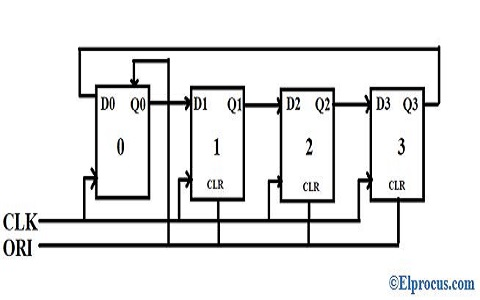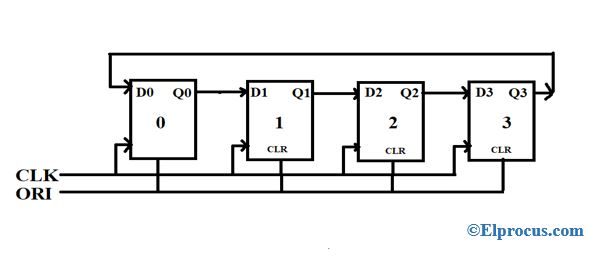# What is a Ring Counter : Working, Classification & Applications

Counters are sequential circuits whose function is to count pulse, frequency and time of the signal using a single clock signal. It is an important component of digital electronics since entire electronic devices work on counters. They are designed by grouping a (similar or different) set of flipflops. Counters operate in different modes of modules, which are represented by the number of states of the cycle. There are two types of counters, they are synchronous and asynchronous counter. The synchronous counter operates based on the input clock signal and the asynchronous counter is independent of the input clock signal. The synchronous counter is a shift register counter which is further classified as a ring-type and twisted type ring counter.

## What is Ring Counter?

Definition: A ring counter is also known as SISO (serial in serial out) shift register counter, where the output of the flip flop is connected to the input of the flip flop which acts as a ring counter. The designing of the ring counter can be done by using four D-Flip Flops with a common clock signal and overriding input can be connected to pre-set and clear.block-diagram-of-ring-counter

From the above diagram,

1). The number of states used is 4 (Where no of states = no of flip flops used).

2). Pre-set or Clear: The main function of this is if the input clock signal changes then the output value is also changed.

The connections are made as follows

• One input is connected to the first flip-flop ff0-Q0,
• Another input is connected to CLR of the other three flip flops like ff1, ff2, ff3.

### Working Theory

For example, let us take a condition where pre-set = ‘0000’ then the outputs obtained at each flip flop is as follows. For FF0, the output at Q0 is ‘1’, whereas in other flipflops like ff, ff2, ff3 (which are connected to clear where CLR = 0) the outputs obtained at Q1 = Q2 = Q3 =’0′. This can be understood by following the truth table and its output waveforms obtained when executed using Verilog HDL code in Xilinx software.

#### Truth Table

 ORI CLK Q0 Q1 Q2 Q3 Low Pulse X 1 0 0 0 1 0 0 1 0 0 1 0 0 0 1 0 1 0 0 0 0 1 1 0 1 0 0 0

Where

Inputs = ORI and CLK

X = Clock can be either a positive edge or a negative edge

Outputs = Q0, Q1, Q2, Q3.

From the table, we can observe that ‘1’ is shifted diagonally from Q0 to Q3 and again will shifts back to ‘Q0’. So this shows that it works like a ring counter.

### Verilog HDL Program for Ring Counter

module dff(q,d,c);
output q;
input d,c;
reg q;
initial
q=1’b1;
always @ (posedge c)
q=d;
end module

module dff1(q,d,clk);
output q;
input d,clk;
reg q;
initial
q=1’b0;
always @ (posedge clk)
q=d;
endmodule

module ring(q,clk);
inout [3:0]q;
input clk;
dff u1(q,q,clk);
dff1 u2(q,q,clk);
dff1 u3(q,q,clk);
dff1 u4(q,q,clk);
end module

#### Timing Diagram of Ring Counter

The timing diagram of the ring counter is shown below.

### Classification of Ring Counters

Ring counters are classified into two they are,

#### Straight Type

The alternative name of a straight type is ‘one hot counter’, where the output of ending flip flop is given as a feedback to the input of starting flip flop. Where binary digit 0/1 is circulated in ring form. Two control signals Pre-set (PR) and the clock signal (CLK) are used. Where PR is connected to FF 0 and CLR is given to FF3. The following is the block diagram of 4 stages straight ring counter.

#### Twisted Type

The alternative name of the twisted type is switch tail/walking/Johnson type counter. The complemented output of ending flip flop is feedback to the input of starting flip flop. Where the stream of 1’s and 0’s flow in ring form. The twisted type counter uses two control signals like CLK and ORI. Where CLK and ORI are common to all four flip flops. The following is the block diagram of 4 stages twisted ring-type counter.#### Truth Table of Twisted Type

 ORI CLK Q0 Q1 Q2 Q3 Low Pulse X 0 0 0 0 1 1 1 0 0 0 1 1 1 1 0 0 1 1 1 1 1 0 1 1 1 1 1 1 1 1 0 1 1 1 1 1 0 0 1 1 1 1 0 0 0 1

#### Timing Diagram of Twisted Type

The timing diagram of the twisted type is shown below.

#### Difference between Ring Type Counter and Johnson Type Counter

The following are the comparison between ring counter and Johnson counter

 Ring Counter Johnson Counter The output of the last flipflop is given as input to starting flip flop. The output of the last flip-flop is complemented and given as input to starting flip flop. Number of states = Number of flip flops used If ‘n’ number of flip flops are used then ‘2n’ number of states is required. Input frequency = n Input frequency = f Output frequency = f/n Output frequency = f/2n Total unused states = ( 2n – n) Total unused states = ( 2n – 2n)

• It can encode and decode the logics
• Implementation can be done using JK and D flip flops

• Out of 15 states, 4 states are used
• Non-self-starting.

#### Applications

The following are the applications

### FAQ’s

1). How many states are there in the 10-bit ring counter?

10 states are used in the 10-bit ring counter.

2). What is the asynchronous counter?

An asynchronous counter operates asynchronously that is it is independent on clock pulse. It has 2n – 1 states.

3). What is a mod of a counter?

Another name for a mod counter is the Modulus counter. It is defined as the number of states in a counter.

4). What do you mean by Johnson’s counter?

Johnson counter is one type of ring counter, where the output of the last flip-flop is complemented and feedback to the input of the first flip-flop. The number of states used is 2n.

5). What is a divide by N counter?

Divided by N counter means the division of input clock frequency by N.

6). What do you mean by the SISO shift register?

A SISOshift register is a serial in-serial out the register, where the input data and output data is processed serially one after the other and the result is stored in the register.

Thus, a counter is an important component of digital electrons. They are classified as synchronous(ring-type and twisted type) and asynchronous counters. Thus, this is an overview of a ring counter which uses two control signals, clock and pre-set. Based on these signals they operate in ring format hence it is called a ring counter, they are further classified as a straight and twisted type. Where each counter has its own design, advantages, and disadvantages.